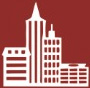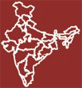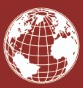ctaforum@gmail.com +91-9810447742, +91-9953805381

### Registration

Educator School

Educator School

1. Under which major heading “Unclaimed Dividend” appears in the Balance Sheet of a Company.

(a) Current Assets

(b) Non Current Liabilities

(c) Current liabilities and Provisions

(d) Share holders fund

Ans: (c) Current liabilities and Provisions

2. Investments which are necessary for the operations of the business enterprise are called

(a) Long Term Investments         (b) Short Term Investments

3. If Quick ratio is 2 : 1. State the effect of purchase goods on credit for ₹ 2,000

(a) Improve                                        (b) Reduce

(c) No Effect                                       (d) May be improve or no effect

Ans: (b) Reduce

4. Dividend is usually paid on:

(a) Authorised Capital                    (b) Issued Capital

(c) Paid-up Capital                           (d) Uncalled Capital

Ans:  (c) Paid-up Capital

5. Working Capital Ratio is calculated by the formula:

(a) Current Assets/Current liabilities

(b) Net working capital/Net Sales

(c) Net working capital/Fixed Assets

(d) Working capital/Capital Employed

Ans: (a) Current Assets/Current liabilities

6. Which ratio indicates the proportion of total assets financed by shareholders Funds.

(a) Total Assets to Debt Ratio     (b) Fixed Assets turnover Ratio

(c) Proprietary Ratio                       (d) Debt Equity Ratio

Ans: (c) Proprietary Ratio

7. Horizontal analysis is:

(a) The calculation of relative weighting of components within a particular financial period.

(b) The comparison of the current year’s figures with the previous year’s figures.

(c) The comparison of one company’s results with another company.

(d) The comparison of the profit and loss account with the balance sheet.

Ans: (b) The comparison of the current year’s figures with the previous year’s figures.

8. Which Ratio measure the firm’s ability to meet its short term obligations.

(a) Liquidity Ratios.                          (b) Liquid Ratio

(c) Solvency ratios                           (d) Current Ratio

Ans:       (a) Liquidity Ratios.

9. The Debt Equity Ratio from the following information will be:

Total Assets                        ₹ 5,30,000

Current Liabilities             ₹ 1,80,000

Total Debts                         ₹ 3,30,000

(a) 0.75 : 1                                           (b) 0.77 : 1

(c) 0.67 : 1                                            (d) 0.73 : 1

Ans: (a) 0.75 : 1

10. What will be the sales from the following particulars Opening Stock ₹ 4,00,000

Inventory Turnover Ratio             6 Times

Gross Profit                                        20% on sales

Further Closing stock is twice the opening stock.

(a) ₹ 4,50,000                                     (c) ₹ 3,60,000

(b) ₹ 2,88,000                                     (d) ₹ 4,80,0000

Ans: (a) ₹ 4,50,000

11. Match columns-I and columns-II:

Column-I                                           Column-II

(i) Employees                    A. Interested in knowing the profitability and financial strength of the enterprise

(ii) Creditors                       B. Interested in knowing the progress of the enterprise to ensure the safety and                  recovery of the loan advanced

(iii) Owners                         C. Interested in knowing the ability of the enterprise to provide remuneration, retirement benefits and employment opportunities.

(iv) Banks and Financial Enterprise                           D. Interested in knowing about the credit worthiness of the business.

Select from the following options:

(a) (i)-B; (ii)-D; (iii)-A; (iv)-C       (b) (i)-C; (ii)-A; (iii)-D; (iv)-B

(c) (i)-C; (ii)-D; (iii)-A; (iv)-B       (d) (i)-C; (ii)-D; (iii)-B; (iv)-A

Ans: (c) (i)-C; (ii)-D; (iii)-A; (iv)-B

12. If current ratio of a company is 2 : 1. What will be the effect on this ratio when current assets increases by ₹ 50,000 and current liabilities increases by ₹ 40,000.

(a) The current ratio will increase

(b) The current ratio will decrease.

(c) There will be no change in current ratio.

(d) The ratio increased to 2.5 : 1.

Ans: (b) The current ratio will decrease

13. The proprietary ratio of a firm is 60%. Which of the following transactions would not result in increase in this ratio?

(a) Issue of preference shares

(b) Conversion of debentures into preference shares.

(c) Redemption of debentures

(d) Purchase of goods on credit

Ans: (d) Purchase of goods on credit

14. The Interest coverage ratio from the following information will be:

General Reserve                                              ₹ 45,000,

Share Capital                                                      ₹ 2,00,000,

15% Loan                                                             ₹ 1,00,000

Sales for the year                                             ₹ 2,50,000

Tax paid during the year                               ₹ 40,000

Profit after interest and tax                         ₹ 80,000

(a) 9 Times                          (b) 6 Times

(c) 5 Times                          (d) 8 Times

Ans: (a) 9 Times

15. The Operating profit ratio from the following information will be:

Selling and distribution expenses             ₹ 40,000

Interest paid on debentures                       ₹ 9,000

Net Profit                                                            ₹ 71,000

Net Sales                                                             ₹ 5,00,000

(a) 54%                                                 (b) 5.4%

(c) 15%                                                 (d) 4.4%

Ans: (c) 15%

16. Where will ‘Loose tools' be shown in the Balance Sheet of a company as per revised schedule III:

17. In common size statement of Profit and Loss is taken as base figure for calculation of percentages.

(a) Total Revenue                            (b) Profit before Tax

(c) Revenue from operations     (d) Total Assets

Ans: (c) Revenue from operations

18. Current ratio of a company is 2.5 : 1 and its Quick ratio is 1.5 : 1. Payment of dividend already declared would result in:

(a) Increase in Current Ratio and Quick Ratio

(b) Decrease in Current Ratio and Quick Ratio

(c) No effect on Current Ratio

(d) No effect on Quick Ratio

Ans: (b) Decrease in Current Ratio and Quick Ratio

19. Which ratio indicates the proportion of total assets financed by shareholders funds?

(a) Total Assets to Debt Ratio     (b) Fixed Assets turnover Ratio

(c) Proprietary Ratio                       (d) Debt equity Ratio

Ans: (c) Proprietary Ratio

20. What will be the gross profit from the following particulars Opening Stock ₹ 40,000 Inventory turnover Ratio 6 times Gross profit 20% on revenue from operations. Additional Information: Closing stock is twice the opening stock.

(a) ₹ 90,000                                        (b) ₹ 3,60,000

(c) ₹ 4,50,000                                     (d) ₹ 372,000

Ans: (a) ₹ 90,000

21. Working capital turnover ratio is calculated by the formula:

(a) Revenue from operations/Working Capital

(b) Net Working Capital/Revenue from operations

(c) NetWorking Capital/Fixed Assets

(d) Current Assets/Current Liabilities

Ans: (a) Revenue from operations/Working Capital

22. is included in current assets while preparing balance sheet as per

revised Schedule III but excluded from current assets while calculating Current Ratio

(a) Debtors

(b) Cash and Cash Equivalent

(c) Loose tools and Stores and spares

(d) Bank Balance

Ans: (c) Loose tools and Stores and spares

23. Which of the following is not a limitation of Financial Statement Analysis?

(a) Ignores price level changes   (b) Qualitative aspect is ignored

(c) Window Dressing                      (d) Judging Managerial efficiency

Ans: (d) Judging Managerial efficiency

24. If Quick ratio is 3 : 1, What will be the effect on quick ratio in case of purchase of machinery on credit basis for two months?

(a) Improve                                          (b) Reduce

(c) Depends upon Current Assets (d) No effect.

Ans: (b) Reduce

25. What ratio can be used to avoid inefficient buying habits?

(a) Working Turnover Ratio         (b) Gross Profit Ratio

(c) Debt Equity Ratio                       (d) Inventory Turnover Ratio

Ans: (d) Inventory Turnover Ratio

26. measures a firm’s ability to pay all of its fixed charges or expenses

with its income before interest and income tax

(a) Return on investment             (b) Current ratio

(c) Quick ratio                                    (d) Interest Coverage Ratio

Ans: (d) Interest Coverage Ratio

27. If Operating Cycle is 8 months and any amount receivable in 11 months will be categorized as

(a) Current Assets                           (b) Non-urrent Assets

(c) Fictitious Assets                         (d) Intangible Assets

Ans: (a) Current Assets

28. Following sub-headings are to be shown in the balance sheet of the company.

(i) Cash and Cash Equivalents     (ii) Other Current Assets

(iii) Inventories                                 (iv) Current Investment

What will be the correct order of showing them in the books?

(a) (i), (iii), (iv), (v), (ii)                   (b) (iii), (iv), (i), (ii), (v)

(c) (iv), (iii), (v), (i), (ii)                    (d) (iv), (v), (i), (iii), (ii)

Ans: (c) (iv), (iii), (v), (i), (ii)

29. Identify the correct match:

A. Debt                                                 (i) Opening Inventory + Net Purchases + Direct Expenses – Closing Inventory

B. Equity                                              (ii) Shareholder’s Fund + Long term Debts

C. Capital Employed                        (iii) Share Capital + Reserves and Surplus

D. Cost of Revenue from              (iv) Total Debt – Current Liabilities Operations

Choose the correct option:

(a) A-(ii), B-(iii), C-(iv), D-(i)          (b) A-(iv), B-(ii), C-(iii), D-(i)

(c) A-(iv), B-(iii), C-(ii), D-(i)          (d) A-(ii), B-(iv), C-(i), D-(iii)

Ans: (c) A-(iv), B-(iii), C-(ii), D-(i)

30. The current ratio of a XYZ Ltd is 1. What will be its working capital?

(a) 1                                                       (b) 0

(c) 100                                                   (d) None

Ans: (b) 0

31. Debt-Equity Ratio of Dhamaka Ltd is 3 : 1. Which of the following will result in decline in this ratio?

(a) Issue of Debentures for Cash of ₹ 2,00,000.

(b) Issue of Debentures of ₹ 3,00,000 to Vendors from whom Machinery was purchased.

(c) Goods purchased on Credit of ₹ 1,00,000.

(d) Issue of Equity Shares of ₹ 2,00,000.

Ans: (d) Issue of Equity Shares of ₹ 2,00,000.

32. Shareholders’ Funds doesn’t include               from the following

(a)Share Capital

(b) Share Application Money Pending Allotment

(c) Money Received against Share Warrants

(d) Reserves and Surplus

Ans: (b) Share Application Money Pending Allotment

33. Pick the odd one out.

(a) Share Capital                               (b) Reserves and Surplus

(c) Non-Trade Investment           (d) Long Term Debts

34. Match the following:

Column-I                             Column-II

(i) Loose Tools                                   (a) Intangible Fixed Assets

(ii) Mastheads and Publishing     (b) Other Current Assets Titles

(iii) Pre-paid Insurance                  (c) Short term Provisions

(iv) Provision for tax                       (d) Inventories Choose the correct option:

(a) (i)-(a); (ii)-(c); (iii)-(d); (iv)-(d)

(b) (i)-(d); (ii)-(a); (iii)-(b); (iv)-(c)

(c) (i)-(b); (ii)-(d); (iii)-(a); (iv)-(c)

(d) (i)-(c); (ii)-(a); (iii)-(b); (iv)-(d)

Ans: (b) (i)-(d); (ii)-(a); (iii)-(b); (iv)-(c)

35. Which of the following will improve the Current Ratio if Current Ratio is 0.8 : 1

(a) Sales of Goods on Credit costing ₹ 20,000 for ₹ 18,000

(b) ₹ 20,000 paid to Creditors

(c) Issue of shares to vendors against Purchase of Machinery

(d)  Purchase of goods costing ₹ 20,000 on credit.

Ans: (d) Purchase of goods costing ₹ 20,000 on credit.

36.  In a common size statement, % shown against Employee Benefit expenses is 25% and its amount is ₹ 4,00,000. What is Revenue from Operations for this organisation?

(a) ₹ 1,00,000                                     (b) ₹ 20,00,000

(c) ₹ 16,00,000                                   (d) Inadequate Information

Ans:       (c) ₹ 16,00,000

37. From the following information, compute Debt-Equity Ratio

Long term Borrowings                   ₹ 2,00,000;

Long term Provisions                      ₹ 1,00,000;

Shareholder Funds                          ₹ 1,20,000;

Reserves and Surplus                     ₹ 30,000;

Current Liabilities                             ₹ 50,000.

(a) 2 : 1                                 ( b) 2.5 : 1

(c) 2.3 : 1                              (d) 2.9 : 1

37.          (b)

38. Classify the following as a type of liability: Trade payable expected to be paid in 14 months and the operating cycle is of 19 months :

(a) Current Liability          (b) Non-Current Liability

(c) Either (a) or (b)           (d) None of the above

Ans: (a) Current Liability

39. Under which main head Deferred Tax Liability is shown in the Balance Sheet as per the Companies Act 2013?

(a) Non-Current Liability

(b) Current Liability

(c) Shareholders Funds

(d) Share Application Money pending Allotment

Ans: (a) Non-Current Liability

40. Which two Current Assets are not taken into consideration while calculating Current Assets for determining Current Ratio:

(a) Loose tools, stores and spares

(b) Prepaid expenses, Closing stock

(c) Both of (a) and (b)

(d) None of (a) or (b)

Ans: (a) Loose tools, stores and spares

41. Which ratio is used to determine the efficiency of people engaged in debt collection department:

(a) Debt Equity Ratio                      (b) Trade Receivables’ Turnover Ratio

(c) Total Assets to Debt Ratio      (d) Proprietary Ratio

Ans: (b) Trade Receivables’ Turnover Ratio

42. If profit after interest and tax is ₹ 1,68,000, calculate profit before interest and tax if tax rate is 40% and interest amount is ₹ 70,000:

(a) ₹ 3,05,200                                     (b) ₹ 3,50,000

(c) ₹ 58,800                                         (d) ₹ 1,70,800

Ans: (b) ₹ 3,50,000

43. Capital employed is equal to:

(a) Equity + Debt

(b) Fixed Assets + Current Assets – Current Liabilities

(c) Fixed Assets + Working Capital

(d) All of the above

Ans: (a) Equity + Debt

44. In a Common Size Balance Sheet of ABC Ltd. Current Assets amounted to ₹ 20,00,000 and it is mentioned as 40% in the statement. If Current Liabilities are ₹ 15,00,000, then what should be the Percentage specified in front of Current Liabilities:

(a) 75%                                 (b) 133.3%

(c) 30%                                 (d) Incomplete Information

Ans: (c) 30%

•106+Cities
•2,00,000+Students
•1000+Schools
•22+States
•6+Countries